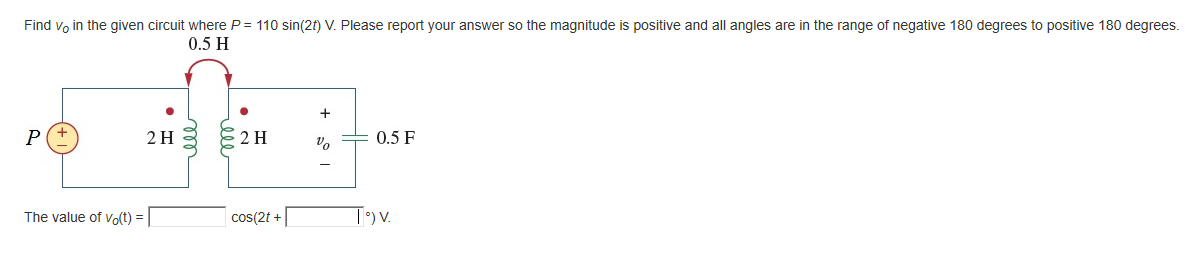# Question Find Voin the given circuit where P = 110 sin(21) V. Please report your answer so the magnitude is positive and all angles are in the range of negative 180 degrees to positive 180 degrees. 0.5 H ... P + 2 H ੩ 2 H - 0.5 F The value of Vo(t) = cos(2t + To).MWG7E6 The Asker · Electrical EngineeringTranscribed Image Text: Find Voin the given circuit where P = 110 sin(21) V. Please report your answer so the magnitude is positive and all angles are in the range of negative 180 degrees to positive 180 degrees. 0.5 H ... P + 2 H ੩ 2 H - 0.5 F The value of Vo(t) = cos(2t + To).
More
Transcribed Image Text: Find Voin the given circuit where P = 110 sin(21) V. Please report your answer so the magnitude is positive and all angles are in the range of negative 180 degrees to positive 180 degrees. 0.5 H ... P + 2 H ੩ 2 H - 0.5 F The value of Vo(t) = cos(2t + To).